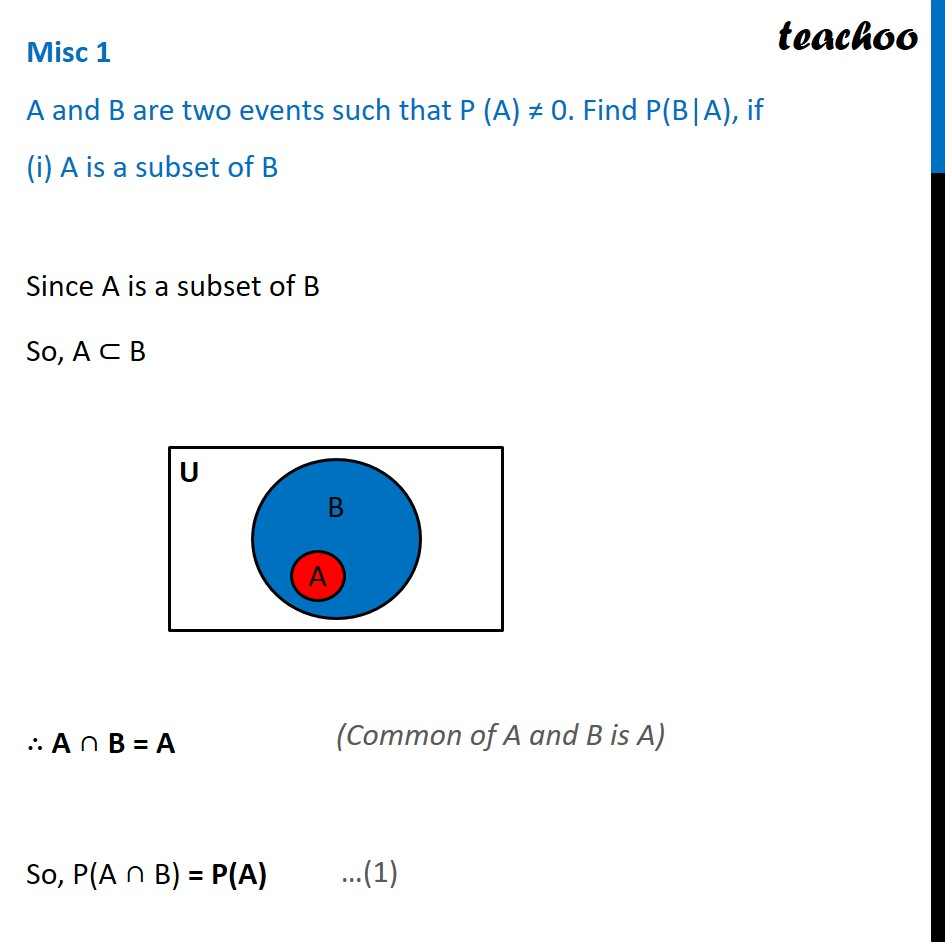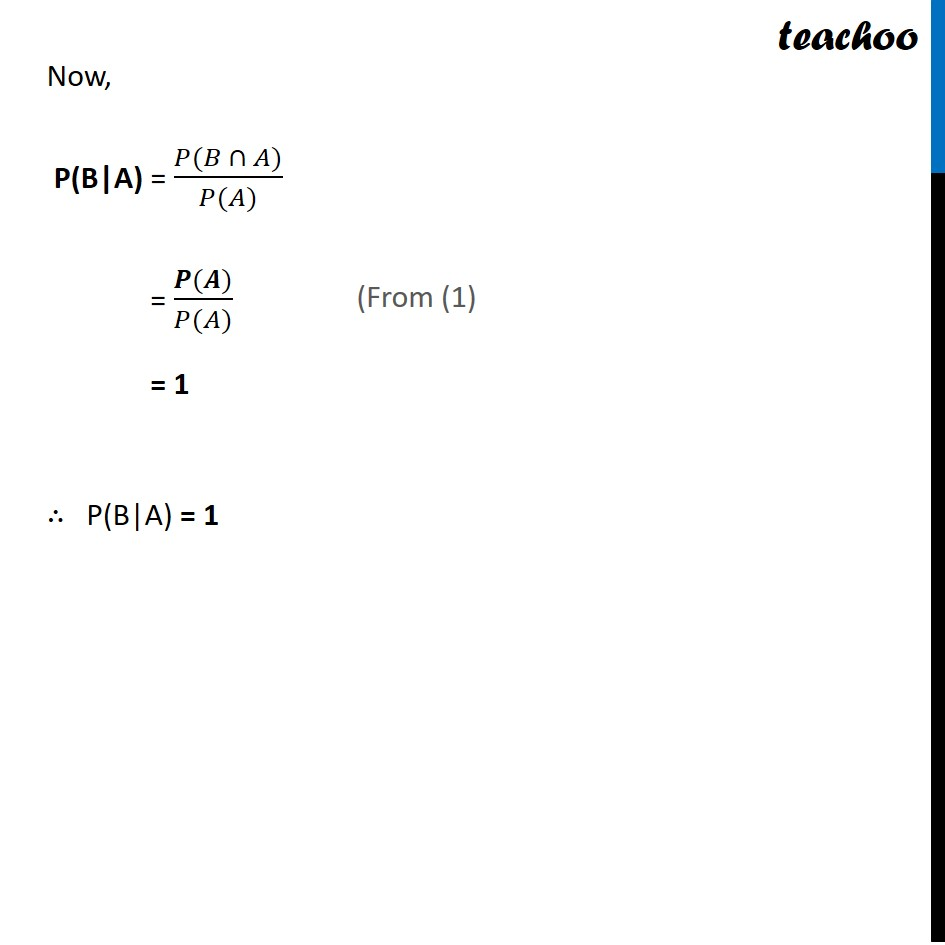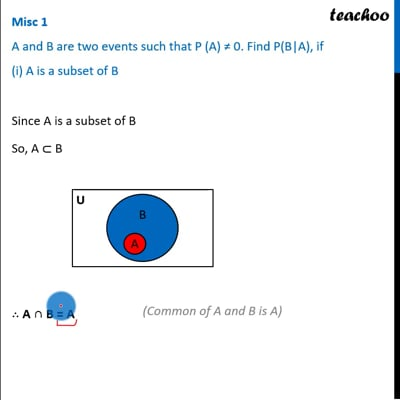Conditional Probability - Values given

Chapter 13 Class 12 Probability
Concept wiseThis video is only available for Teachoo black users

Introducing your new favourite teacher - Teachoo Black, at only ₹83 per month

### Transcript

Misc 1 A and B are two events such that P (A) 0. Find P(B|A), if (i) A is a subset of B Since A is a subset of B So, A B A B = A So, P(A B) = P(A) Now, P(B|A) = ( ) ( ) = ( ) ( ) = ( ) ( ) = 1 P(B|A) = 1